This documentation is for scikit-learn version 0.11-gitOther versions

### Citing

If you use the software, please consider citing scikit-learn.

# 3.4. Nearest Neighbors¶

sklearn.neighbors provides functionality for unsupervised and supervised neighbors-based learning methods. Unsupervised nearest neighbors is the foundation of many other learning methods, notably manifold learning and spectral clustering. Supervised neighbors-based learning comes in two flavors: classification for data with discrete labels, and regression for data with continuous labels.

The principle behind nearest neighbor methods is to find a predefined number of training samples closest in distance to the new point, and predict the label from these. The number of samples can be a user-defined constant (k-nearest neighbor learning), or vary based on the local density of points (radius-based neighbor learning). The distance can, in general, be any metric measure: standard Euclidean distance is the most common choice. Neighbors-based methods are known as non-generalizing machine learning methods, since they simply “remember” all of its training data (possibly transformed into a fast indexing structure such as a Ball Tree or KD Tree.).

Despite its simplicity, nearest neighbors has been successful in a large number of classification and regression problems, including handwritten digits or satellite image scenes. It is often successful in classification situations where the decision boundary is very irregular.

The classes in sklearn.neighbors can handle either Numpy arrays or scipy.sparse matrices as input. It currently supports only the Euclidean distance metric.

## 3.4.1. Unsupervised Nearest Neighbors¶

NearestNeighbors implements unsupervised nearest neighbors learning. It acts as a uniform interface to three different nearest neighbors algorithms: BallTree, scipy.spatial.cKDTree, and a brute-force algorithm based on routines in sklearn.metrics.pairwise. The choice of neighbors search algorithm is controlled through the keyword 'algorithm', which must be one of ['auto', 'ball_tree', 'kd_tree', 'brute']. When the default value 'auto' is passed, the algorithm attempts to determine the best approach from the training data. For a discussion of the strengths and weaknesses of each option, see Nearest Neighbor Algorithms.

## 3.4.2. Nearest Neighbors Classification¶

Neighbors-based classification is a type of instance-based learning or non-generalizing learning: it does not attempt to construct a general internal model, but simply stores instances of the training data. Classification is computed from a simple majority vote of the nearest neighbors of each point: a query point is assigned the data class which has the most representatives within the nearest neighbors of the point.

scikit-learn implements two different nearest neighbors classifiers: KNeighborsClassifier implements learning based on the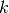nearest neighbors of each query point, whereis an integer value specified by the user. RadiusNeighborsClassifier implements learning based on the number of neighbors within a fixed radius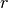of each training point, whereis a floating-point value specified by the user.

The-neighbors classification in KNeighborsClassifier is the more commonly used of the two techniques. The optimal choice of the valueis highly data-dependent: in general a largersuppresses the effects of noise, but makes the classification boundaries less distinct.

In cases where the data is not uniformly sampled, radius-based neighbors classification in RadiusNeighborsClassifier can be a better choice. The user specifies a fixed radius, such that points in sparser neighborhoods use fewer nearest neighbors for the classification. For high-dimensional parameter spaces, this method becomes less effective due to the so-called “curse of dimensionality”.

The basic nearest neighbors classification uses uniform weights: that is, the value assigned to a query point is computed from a simple majority vote of the nearest neighbors. Under some circumstances, it is better to weight the neighbors such that nearer neighbors contribute more to the fit. This can be accomplished through the weights keyword. The default value, weights = 'uniform', assigns uniform weights to each neighbor. weights = 'distance' assigns weights proportional to the inverse of the distance from the query point. Alternatively, a user-defined function of the distance can be supplied which is used to compute the weights.

Examples:

## 3.4.3. Nearest Neighbors Regression¶

Neighbors-based regression can be used in cases where the data labels are continuous rather than discrete variables. The label assigned to a query point is computed based the mean of the labels of its nearest neighbors.

scikit-learn implements two different neighbors regressors: KNeighborsRegressor implements learning based on thenearest neighbors of each query point, whereis an integer value specified by the user. RadiusNeighborsRegressor implements learning based on the neighbors within a fixed radiusof the query point, whereis a floating-point value specified by the user.

The basic nearest neighbors regression uses uniform weights: that is, each point in the local neighborhood contributes uniformly to the classification of a query point. Under some circumstances, it can be advantageous to weight points such that nearby points contribute more to the regression than faraway points. This can be accomplished through the weights keyword. The default value, weights = 'uniform', assigns equal weights to all points. weights = 'distance' assigns weights proportional to the inverse of the distance from the query point. Alternatively, a user-defined function of the distance can be supplied, which will be used to compute the weights.

Examples:

## 3.4.4. Nearest Neighbor Algorithms¶

### 3.4.4.1. Brute Force¶

Fast computation of nearest neighbors is an active area of research in machine learning. The most naive neighbor search implementation involves the brute-force computation of distances between all pairs of points in the dataset: for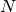samples in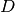dimensions, this approach scales as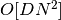. Efficient brute-force neighbors searches can be very competetive for small data samples. However, as the number of samplesgrows, the brute-force approach quickly becomes infeasible. In the classes within sklearn.neighbors, brute-force neighbors searches are specified using the keyword algorithm = 'brute', and are computed using the routines available in sklearn.metrics.pairwise.

### 3.4.4.2. K-D Tree¶

To address the computational inefficiencies of the brute-force approach, a variety of tree-based data structures have been invented. In general, these structures attempt to reduce the required number of distance calculations by efficiently encoding aggregate distance information for the sample. The basic idea is that if point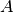is very distant from point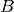, and pointis very close to point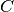, then we know that pointsandare very distant, without having to explicitly calculate their distance. In this way, the computational cost of a nearest neighbors search can be reduced to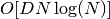or better. This is a significant improvement over brute-force for large.

An early approach to taking advantage of this aggregate information was the KD tree data structure (short for K-dimensional tree), which generalizes two-dimensional Quad-trees and 3-dimensional Oct-trees to an arbitrary number of dimensions. The KD tree is a tree structure which recursively partitions the parameter space along the data axes, deviding it into nested orthotopic regions into which data points are filed. The construction of a KD tree is very fast: because partitioning is performed only along the data axes, no-dimensional distances need to be computed. Once constructed, the nearest neighbor of a query point can be determined with only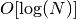distance computations. Though the KD tree approach is very fast for low-dimensional (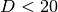) neighbors searches, it becomes inefficient asgrows very large: this is one manifestation of the so-called “curse of dimensionality”. In scikit-learn, KD tree neighbors searches are specified using the keyword algorithm = 'kd_tree', and are computed using the class scipy.spatial.cKDTree.

References:

### 3.4.4.3. Ball Tree¶

To address the inefficiencies of KD Trees in higher dimensions, the ball tree data structure was developed. Where KD trees partition data along cartesian axes, ball trees partition data in a series of nesting hyper-spheres. This makes tree construction more costly than that of the KD tree, but results in a data structure which allows for efficient neighbors searches even in very high dimensions.

A ball tree recursively divides the data into nodes defined by a centroidand radius, such that each point in the node lies within the hyper-sphere defined byand. The number of candidate points for a neighbor search is reduced through use of the triangle inequality: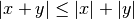With this setup, a single distance calculation between a test point and the centroid is sufficient to determine a lower and upper bound on the distance to all points within the node. Because of the spherical geometry of the ball tree nodes, its performance does not degrade at high dimensions. In scikit-learn, ball-tree-based neighbors searches are specified using the keyword algorithm = 'ball_tree', and are computed using the class sklearn.neighbors.BallTree. Alternatively, the user can work with the BallTree class directly.

References:

### 3.4.4.4. Choice of Nearest Neighbors Algorithm¶

The optimal algorithm for a given dataset is a complicated choice, and depends on a number of factors:

• number of samples(i.e. n_samples) and dimensionality(i.e. n_features).

• Brute force query time grows as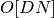• Ball tree query time grows as approximately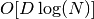• KD tree query time changes within a way that is difficult to precisely characterise. For small(less than 20 or so) the cost is approximately, and the KD tree query can be very efficient. For larger, the cost increases to nearly O[DN], and the overhead due to the tree structure can lead to queries which are slower than brute force.

For small data sets (less than 30 or so),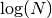is comparable to, and brute force algorithms can be more efficient than a tree-based approach. Both cKDTree and BallTree address this through providing a leaf size parameter: this controls the number of samples at which a query switches to brute-force. This allows both algorithms to approach the efficiency of a brute-force computation for small.

• data structure: intrinsic dimensionality of the data and/or sparsity of the data. Intrinsic dimensionality refers to the dimension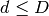of a manifold on which the data lies, which can be linearly or nonlinearly embedded in the parameter space. Sparsity refers to the degree to which the data fills the parameter space (this is to be distinguished from the concept as used in “sparse” matrices. The data matrix may have no zero entries, but the structure can still be “sparse” in this sense).

• Brute force query time is unchanged by data structure.
• Ball tree and KD tree query times can be greatly influenced by data structure. In general, sparser data with a smaller intrinsic dimensionality leads to faster query times. Because the KD tree internal representation is aligned with the parameter axes, it will not generally show as much improvement as ball tree for arbitrarily structured data.

Datasets used in machine learning tend to be very structured, and are very well-suited for tree-based queries.

• number of neighborsrequested for a query point.

• Brute force query time is largely unaffected by the value of• Ball tree and KD tree query time will become slower asincreases. This is due to two effects: first, a largerleads to the necessity to search a larger portion of the parameter space. Second, using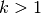requires internal queueing of results as the tree is traversed.

Asbecomes large compared to, the ability to prune branches in a tree-based query is reduced. In this situation, Brute force queries can be more efficient.

• number of query points. Both the ball tree and the KD Tree require a construction phase. The cost of this construction becomes negligible when amortized over many queries. If only a small number of queries will be performed, however, the construction can make up a significant fraction of the total cost. If very few query points will be required, brute force is better than a tree-based method.

Currently, algorithm = 'auto' selects 'ball_tree' if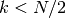, and 'brute' otherwise. This choice is based on the assumption that the number of query points is at least the same order as the number of training points, and that leaf_size is close to its default value of 30.

### 3.4.4.5. Effect of leaf_size¶

As noted above, for small sample sizes a brute force search can be more efficient than a tree-based query. This fact is accounted for in the ball tree and KD tree by internally switching to brute force searches within leaf nodes. The level of this switch can be specified with the parameter leaf_size. This parameter choice has many effects:

construction time
A larger leaf_size leads to a faster tree construction time, because fewer nodes need to be created
query time
Both a large or small leaf_size can lead to suboptimal query cost. For leaf_size approaching 1, the overhead involved in traversing nodes can significantly slow query times. For leaf_size approaching the size of the training set, queries become essentially brute force. A good compromise between these is leaf_size = 30, the default value of the parameter.
memory
As leaf_size increases, the memory required to store a tree structure decreases. This is especially important in the case of ball tree, which stores a-dimensional centroid for each node. The required storage space for BallTree is approximately 1 / leaf_size times the size of the training set.

leaf_size is not referenced for brute force queries.